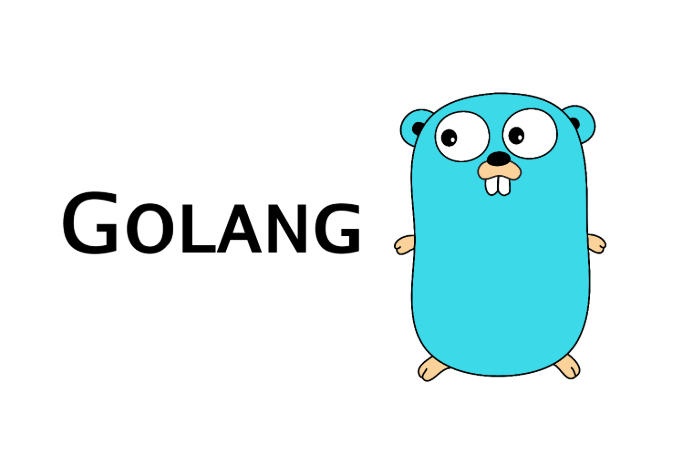# Go语言入门教程(十一)Hello，各位小伙伴大家好，我是小栈君，昨天讲了关于go语言的函数的定义和自定函数的部分种类，我们今天接着上期所讲的内容继续进行分享。

 //方式1
func Test01() int {
return 250
}

//方式2, 给返回值命名
func Test02() (a int) {
a = 250
return a
}
//方式3, 给返回值命名
func Test03() (value int) {
value = 250
return
}

func main() {
v1 := Test01() //函数调用
v2 := Test02() //函数调用
v3 := Test03() //函数调用
fmt.Printf("v1 = %d, v2 = %d, v3 = %d\n", v1, v2, v3)
}

//方式1
func Test01() (int, string) {
return 520, "it干货栈"
}
//方式2, 给返回值命名
func Test02() (a int, str string) {
a = 520
str = "it干货栈"
return
}

func  main() {
v1, v2 := Test01() //函数调用
_, v3 := Test02()  //函数调用， 第一个返回值丢弃
v4, _ := Test02()  //函数调用， 第二个返回值丢弃
fmt.Printf("v1 = %d, v2 = %s, v3 = %s, v4 = %d\n", v1, v2, v3, v4)
}

//求2个数的和差
func SumAndSubtract(num1 int, num2 int) (sun int, subtract int) {

return num1 +num2 ,num1-num2
}

func main() {
min, max := SumAndSubtract(33, 22)
fmt.Printf("min = %d, max = %d\n", min, max)
//sum = 55, subtract = 11
}

// 普通实现，利用循环进行累加
func Test01() int {
i := 1
sum := 0
for i = 1; i <= 100; i++ {
sum += i
}

return sum
}

//通过递归实现1+2+3……+100
func Test02(num int) int {
if num == 1 {
return 1
}
return num + Test02(num-1) //函数调用本身
}

//通过递归实现1+2+3……+100
func Test03(num int) int {
if num == 100 {
return 100
}

return num + Test03(num+1) //函数调用本身
}

func main() {
fmt.Println(Test01())    //5050
fmt.Println(Test02(100)) //5050
fmt.Println(Test03(1))   //5050
}posted @ 2019-10-10 08:57  it_carry  阅读(...)  评论(...编辑  收藏